##### On the Gaussıan and Mean Curvatures of Parallel Hypersurfaces in E₁ⁿ⁺¹

Ayşe Yaşar Yavuz, F. Nejat Ekmekci, Yusuf Yaylı

Journal of Advances in Mathematics and Computer Science, Page 590-596
DOI: 10.9734/BJMCS/2014/5963

Let M be a hypersurfaces in (n+1) dimensional Lorentzian space E₁ⁿ⁺¹ and be a parallel hypersurfaces to M. Before now in  the theorem was proved on M in Euclidean space, but now we prove this theorem on in Lorentzian Space. In this study, we give higher order Gaussian curvatures of M in Lorentzian space by using its principal curvatures and we proved the theorem with induction method by using higher order Gaussian curvatures of M in Lorentzian space.

##### A Nonlocal Boundary Value Mixed Problem for Second-order Hyperbolic-parabolic Equations in Nonclassical Function Spaces

DJIBIBE Moussa Zakari, TCHARIE Kokou

Journal of Advances in Mathematics and Computer Science, Page 597-611
DOI: 10.9734/BJMCS/2014/6691##### Applications of Loop Trees

Ward Douglas Maurer

Journal of Advances in Mathematics and Computer Science, Page 612-666
DOI: 10.9734/BJMCS/2014/5816

Aims: In a previous paper we have obtained a result which provides a new way to consider structured programs. Any directed graph whatsoever, according to this result, is a dag overlaid by a structured graph, with loops within loops in which no loops overlap. In such a structured graph, the only backward edges go from somewhere in a loop to the head of that loop. Crucial to this result is the construction of what we call a loop tree. As suggested by R. E. Tarjan, we here apply this result to three well-known situations. We also compare our decomposition method to two earlier such methods, one by Tarjan and one, much older, by Luce.
Methodology: We use the conventions of classical mathematics, in which sets and functions underlie all structures, such as directed graphs and loop trees, and in which all facts obtained in the course of the work are presented as theorems and lemmas, based on definitions and accompanied by valid proofs.
Results: We give a method of solving the single-source path expression problem for a reducible graph by examining its loop tree, which must be unique. We give a necessary and sufficient loop tree condition for a graph to have two edge-disjoint spanning trees, and a necessary and sufficient loop tree condition for a graph to have a feedback vertex.
Conclusion: The study of loop trees can be used to clarify many situations in the theory of directed graphs, in addition to the complete classification of directed graphs mentioned above.

##### The Fourier Multipliers of p-Fourier Spaces on Compact Groups

E. J. Atto, Y. Mensah, V. S. K. Assiamoua

Journal of Advances in Mathematics and Computer Science, Page 667-673
DOI: 10.9734/BJMCS/2014/5086

In this paper, we prove some properties of Fourier multipliers on compact groups. Mainly we obtain the invariance of p-Fourier spaces under the action of Fourier multipliers over compact groups.

##### Weak RIP and Its Application to Compressed Sensing

Hiroshi Inoue

Journal of Advances in Mathematics and Computer Science, Page 674-684
DOI: 10.9734/BJMCS/2014/7298

The first purpose of this paper is to give a sufficient condition under which A obeys the weak RIP and to evaluate the solution of CS using this result. The second is to show that when an m x n random matrix A satisfies the isotropy property: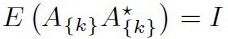for every row vector A{k} of A,always obeys the weak RIP with high probability and it is applicable to the CS theory.

##### A Strong Method for Symmetric Homogeneous Polynomial Inequalities of Degree Six in Nonnegative Real Variables

Vasile Cirtoaje

Journal of Advances in Mathematics and Computer Science, Page 685-703
DOI: 10.9734/BJMCS/2014/7062

Let f6(x, y, z) be a symmetric homogeneous polynomial of degree six. Based on cancelling the high coefficient of f6(x, y, z), we give some practical sufficient conditions to have f6(x, y, z) ≥ 0 for any nonnegative real variables x, y, z. Some applications are given in order to emphasize the effectiveness of the proposed method.

##### A Mathematical Modeling of School Feeding Programme in the Asem – Kumasi Cluster of Schools in Ashanti Region of Ghana

W. Obeng-Denteh, K. A. Gyasi-Agyei, L. Boateng, B. A. Owusu, K. A. Asiedu, O. Antepim

Journal of Advances in Mathematics and Computer Science, Page 704-718
DOI: 10.9734/BJMCS/2014/4949

This paper sought to study and examine the need to investigate with quantitative data whether the objectives of higher enrolment has been met with the introduction of School Feeding Programme in the Ashanti region of Ghana. Time series analysis was used to analyze the data obtained. A best fit model was found by using the Box Jenkin’s approach to time series analysis. Time series and forecasting has been used to model and forecast the enrolment of pupils of schools which were currently enrolled on the Ghana School Feeding Programme with Asem Cluster of Schools as a case study. The forecasted number of pupils who will be enrolled were obtained by using ARIMA (3, 1, 3) model. The AIC for the best selected model was calculated as 1236.17. The study concluded that the school feeding in the region should be continued to attract more pupils.

##### On the Superstability of a Generalization of the Cosine Equation

D. Zeglami, S. Kabbaj, A. Roukbi

Journal of Advances in Mathematics and Computer Science, Page 719-734
DOI: 10.9734/BJMCS/2014/6548

The aim of this paper is to investigate the stability problem for the functional equation:
ƒ(xy)+ƒ(xσ(y))=2g(x)ƒ(y),      x,y∈G                      (Eg,ƒ)
and the superstability of the d'Alembert's equation:
ƒ(xy)+ƒ(xσ(y))=2ƒ(x)ƒ(y),      x,y∈G                      (A)
under the conditions from which the differences of each equation are bounded by φ(x), ψ(x) and min(φ(x),ψ(y)) where G is an arbitrary group, not necessarily abelian, ƒ, g are complex valued functions, φ, ψ are real valued functions and σ is an involution of G.

##### Generalized n-Tupled Common Fixed Point Theorems for Contractive Rational Type Condition

Shamshad Husain, Huma Sahper, Anupam Sharma

Journal of Advances in Mathematics and Computer Science, Page 735-748
DOI: 10.9734/BJMCS/2014/5773

Aims/Objectives: In this paper, we prove results on n-tupled coincidence point (for even n) for a pair of mappings without mixed monotone property satisfying a contractive condition of rational type in partially ordered metric spaces. Our main theorem improves the corresponding results of Chandok et al. (Int. Jour. of Math. Anal., Vol. 7, 2013, No. 9, 433-440).

##### On the Existence and Nonexistence of Positive Solutions for Singular Quasilinear Elliptic Equations with Gradient Terms

Jing Zhang, Zuodong Yang

Journal of Advances in Mathematics and Computer Science, Page 749-758
DOI: 10.9734/BJMCS/2014/6371

In this paper, we investigate the positive solutions of quasilinear elliptic equations of the form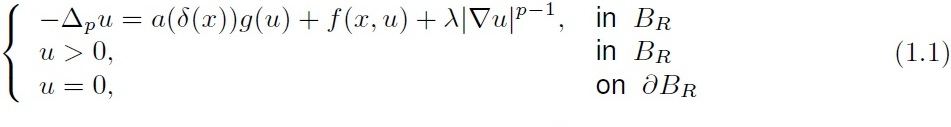where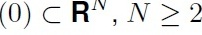is an open ball centered at origin of RN, g is an unbounded decreasing

function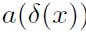is positive and continuous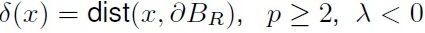. We emphasis the

effect of all these terms in the study of existence and nonexistence of positive solutions.